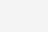# Excel函数技巧检测输入的身份证号码是否合法

• 1.防止输入人员随手输入一个18位数字冒充身份证号码
• 2.防止输入手误,有时候并非故意输错
• 注意本方法无法检测身份证是否真实存在,只能从格式上检查号码是否合法# 身份证号码校验基本原理# 判断身份证号码是否合法的函数公式

=IF(LEN(\$C2)=18,MID(“10X98765432″,MOD(SUMPRODUCT(VALUE(MID(\$C2,ROW(\$1:\$17),1)),\$B\$2:\$B\$18),11)+1,1),”长度错误”)=IF(LEN(\$C2)=18,IF(MID(“10X98765432″,MOD(SUMPRODUCT(VALUE(MID(\$C2,ROW(\$1:\$17),1)),\$B\$2:\$B\$18),11)+1,1)=RIGHT(\$C2,1),”合法”,”不合法”),”长度错误”)# 函数分步讲解

• VALUE(MID(\$C2,ROW(\$1:\$17),1))

ROW函数返回一个1到17的数组，配个mid函数依次取出C2号码中前17个数字，并将截取的文本用value转换为数值

• SUMPRODUCT(VALUE(MID(\$C2,ROW(\$1:\$17),1)),\$B\$2:\$B\$18),11)

• MOD(SUMPRODUCT(VALUE(MID(\$C2,ROW(\$1:\$17),1)),\$B\$2:\$B\$18),11)

MID(“10X98765432”,MOD(SUMPRODUCT(VALUE(MID(\$C2,ROW(\$1:\$17),1)),\$B\$2:\$B\$18),11)+1,1)IF(MID(“10X98765432″,MOD(SUMPRODUCT(VALUE(MID(\$C2,ROW(\$1:\$17),1)),\$B\$2:\$B\$18),11)+1,1)=RIGHT(\$C2,1),”合法”,”不合法”)

IF函数判断计算值是否等于身份证号码第18位，相等则合法，不相等则不合法

=IF(LEN(\$C2)=18,IF(MID(“10X98765432″,MOD(SUMPRODUCT(VALUE(MID(\$C2,ROW(\$1:\$17),1)),\$B\$2:\$B\$18),11)+1,1)=RIGHT(\$C2,1),”合法”,”不合法”),”长度错误”)

=IF(LEN(\$C2)=18,IF(MID(“10X98765432″,MOD(SUMPRODUCT(VALUE(MID(\$C2,ROW(\$1:\$17),1)),\$B\$2:\$B\$18),11)+1,1)=RIGHT(\$C2,1),”合法”,”不合法”),”长度错误”)

(4)

5.1K

25.2K

49.1K

10.3K

4.2K